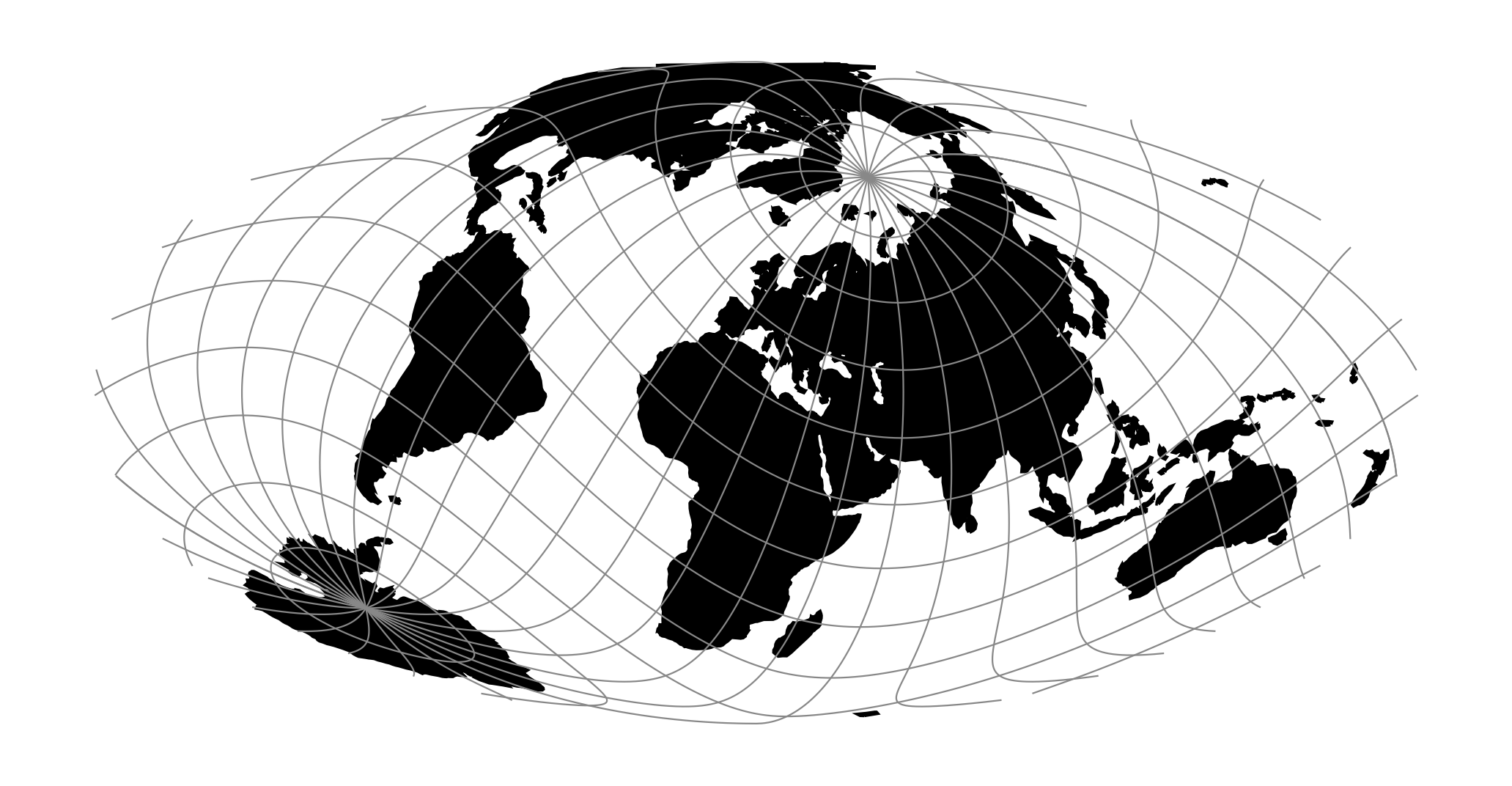# General Oblique Transformation¶proj-string: +proj=ob_tran +o_proj=mill +o_lon_p=40 +o_lat_p=50 +lon_0=60

## Usage¶

All of the projections of spherical library can be used as an oblique projection by means of the General Oblique Transformation. The user performs the oblique transformation by selecting the oblique projection +proj=ob_tran, specifying the translation factors, +o_lat_p, and +o_lon_p, and the projection to be used, +o_proj. In the example of the Fairgrieve projection the latitude and longitude of the pole of the new coordinates, $$\alpha$$ and $$\beta$$ respectively, are to be placed at 45°N and 90°W and use the Mollweide projection. Because the central meridian of the translated coordinates will follow the $$\beta$$ meridian it is necessary to translate the translated system so that the Greenwich meridian will pass through the center of the projection by offsetting the central meridian.

The final control for this projection is:

+proj=ob_tran +o_proj=moll +o_lat_p=45 +o_lon_p=-90 +lon_0=-90


## Parameters¶

### Required¶

+o_proj=<projection>

Oblique projection.

In addition to specifying an oblique projection, how to rotate the projection should be specified. This is done in one of three ways: Define a new pole, rotate the projection about a given point or define a new “equator” spanned by two points on the sphere. See the details below.

#### New pole¶

+o_lat_p=<latitude>

Latitude of new pole for oblique projection.

+o_lon_p=<longitude>

Longitude of new pole for oblique projection.

#### Rotate about point¶

+o_alpha=<value>

Angle to rotate the projection with.

+o_lon_c=<value>

Longitude of the point the projection will be rotated about.

+o_lat_c=<value>

Latitude of the point the projection will be rotated about.

#### New “equator” points¶

+lon_1=<value>

Longitude of first point.

+lat_1=<value>

Latitude of first point.

+lon_2=<value>

Longitude of second point.

+lat_2=<value>

Latitude of second point.

### Optional¶

+lon_0=<value>

Longitude of projection center.

Defaults to 0.0.

+R=<value>

Radius of the sphere given in meters. If used in conjunction with +ellps +R takes precedence.

+x_0=<value>

False easting.

Defaults to 0.0.

+y_0=<value>

False northing.

Defaults to 0.0.